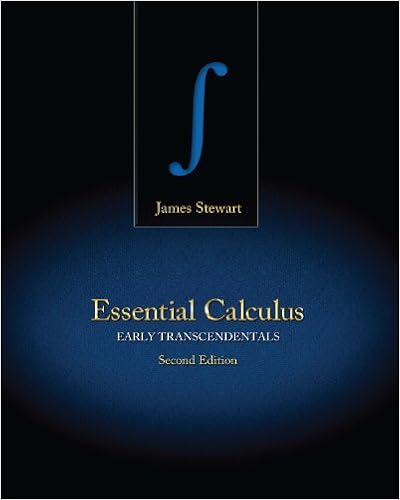# Problem 1 Consider the following ”addition” ⊕ and...

• Notes
• 6

This preview shows page 1 - 3 out of 6 pages.

##### We have textbook solutions for you!
The document you are viewing contains questions related to this textbook.The document you are viewing contains questions related to this textbook.
Chapter 13 / Exercise 18
Essential Calculus: Early Transcendentals
StewartExpert Verified
Math 416 - Practice Exam 1 No calculators, electronic devices, books, or notes may be used. Show your work. No credit for answers without justification. Problem 1. Consider the following ”addition” and ”scalar multiplication” in R 2 . x 1 x 2 y 1 y 2 = x 1 + y 1 + 1 x 2 + y 2 + 1 c x 1 x 2 = cx 1 + c - 1 cx 2 + c - 1 Verify that R 2 is a vector space over R with respect to these operations! Problem 2. Let ( V, + , · ) be a vector space over R . Is it possible to make V a vector space over C using the same addition and a new scalar multiplication defined as ( a + bi ) v := a · v ? Problem 3. Recall that if A = ( a ij ) 2 M n n ( R ), then the trace tr( A ) of A is the sum of the diagonal entries of A , that is tr( A ) = P n i =1 a ii . Show that if A, B 2 M n n ( R ) and c 2 R , then tr( cA + B ) = c tr( A ) + tr( B ). Determine if the subset W consisting of all trace zero matrices in V = M n n ( R ) is a subspace of V . Then give a basis for W and compute the dimension of W . Begin with the case of n = 2. Problem 4. Let X and Y be subspaces of a vector space V . Show that with respect to inclusion, 1) X \ Y is the largest subspace of V contained in both X and Y . 2) X + Y is the smallest subspace of V containing both X and Y . Problem 5. Let V = M 2 2 ( R ) regarded as a vector space over R . For the following subsets X, Y of V , determine if they are subspaces. Then describe X \ Y and X + Y by giving a basis for X , Y , X \ Y , X + Y and computing their dimensions. 1. X = { A 2 V | tr( A ) = 0 } , and Y = diagonal matrices in V . 2. X = ⇢ 0 0 c d | c, d 2 R , and Y = ⇢ 0 a b 0 | a, b 2 R Problem 6. Let X and Y be subspaces of a vector space V . Prove that if X \ Y = { 0 } then dim( X + Y ) = dim( X ) + dim( Y ) What about the case that X \ Y 6 = { 0 } ? Problem 7. Is it possible that vectors v 1 , v 2 , v 3 are linearly dependent, but the vectors v 1 - v 2 , v 2 - v 3 , v 3 - v 1 are linearly independent? 1
##### We have textbook solutions for you!
The document you are viewing contains questions related to this textbook.The document you are viewing contains questions related to this textbook.
Chapter 13 / Exercise 18
Essential Calculus: Early Transcendentals
StewartExpert Verified
Problem 8. Let a 1 , a 2 , a 3 be distinct numbers in R and consider the following polynomials: p 1 ( x ) = ( x - a 2 )( x - a 3 ) , p 2 ( x ) = ( x - a 1 )( x - a 3 ) , p 3 ( x ) = ( x - a 1 )( x - a 2 ) Compute dim R (span { p 1 , p 2 , p 3 } ). Problem 9. What is the dimension of the span of S = { sin x, cos x, sin 2 x, cos 2 x } C ( R , R ) over R ? Problem 10. Determine if the following subsets are subspaces of R 3 . Then give a basis and compute the dimension. Also describe them geometrically.
Problem 11. Let V = P 2 ( R ) and consider the following subsets W of V . Determine if W is a subspace of V , give a basis for W
•••